Calculus II

Day 09-Lecture 18

6 March 2017, Monday 11:40

Previous Screenshot       Next Screenshot

Min & Max of functions of several variables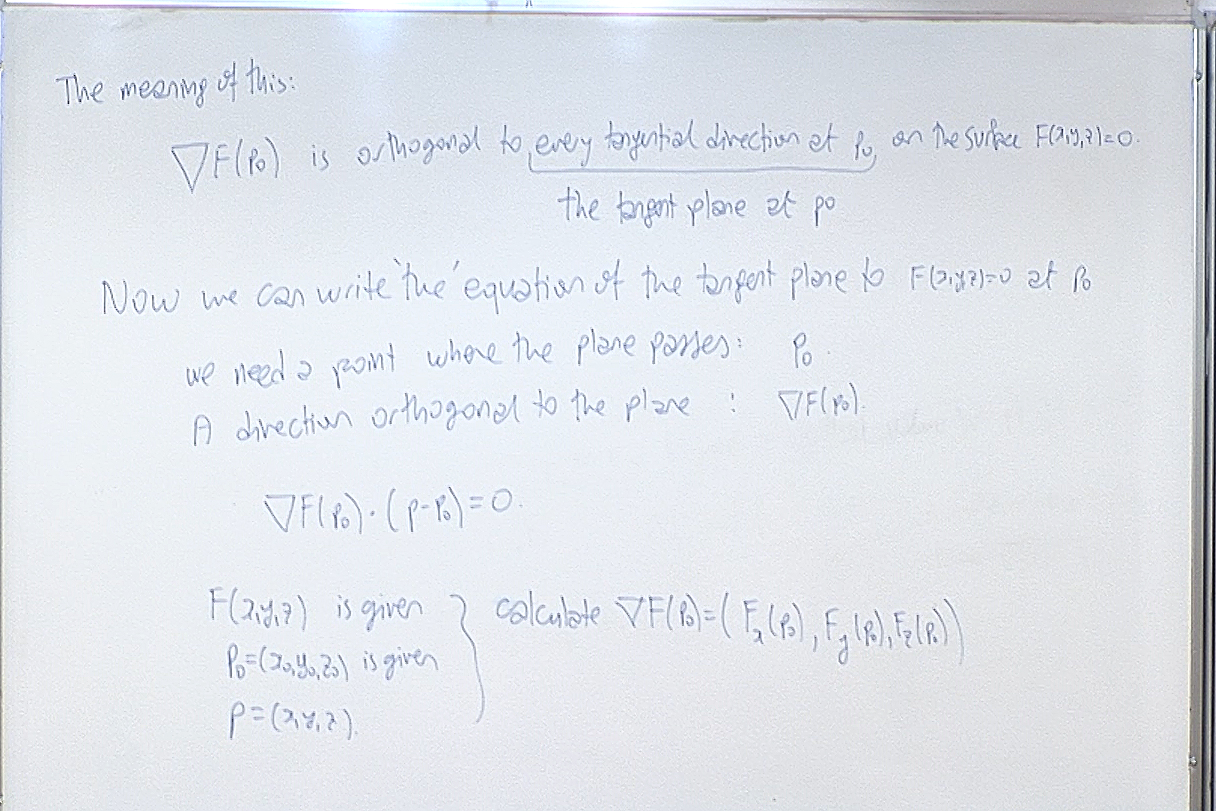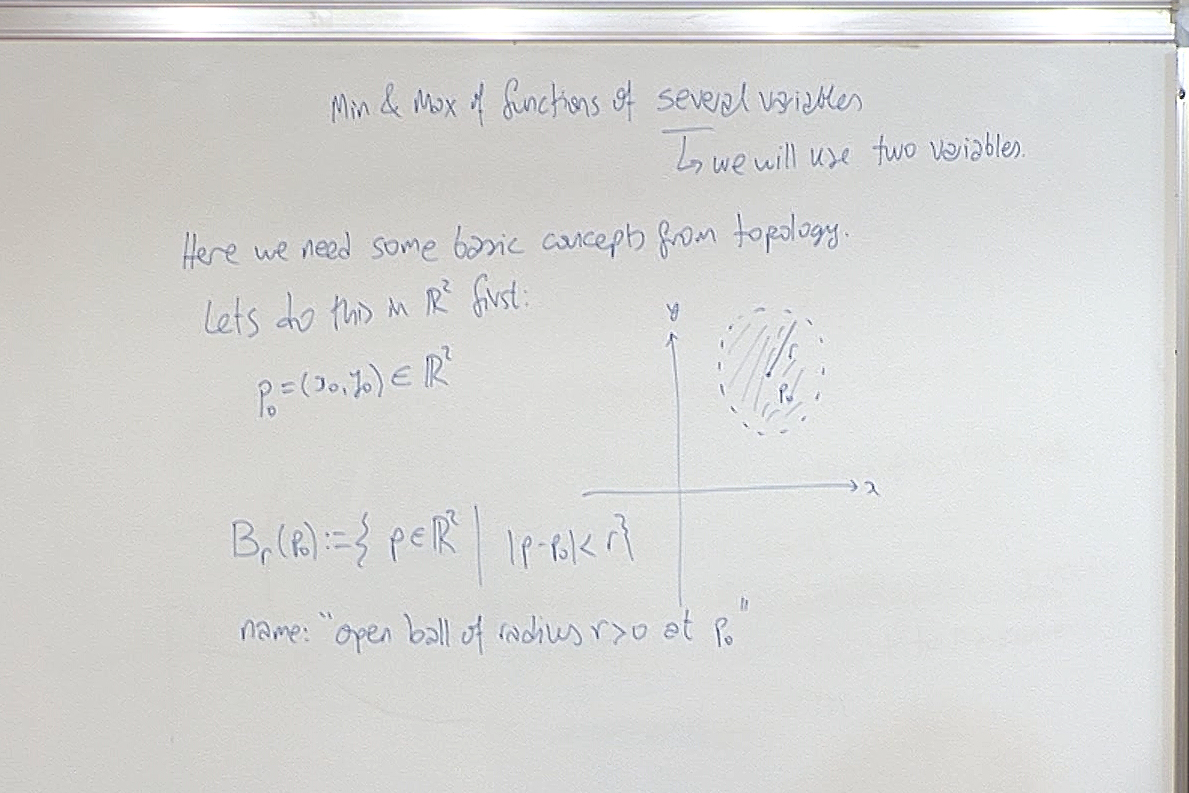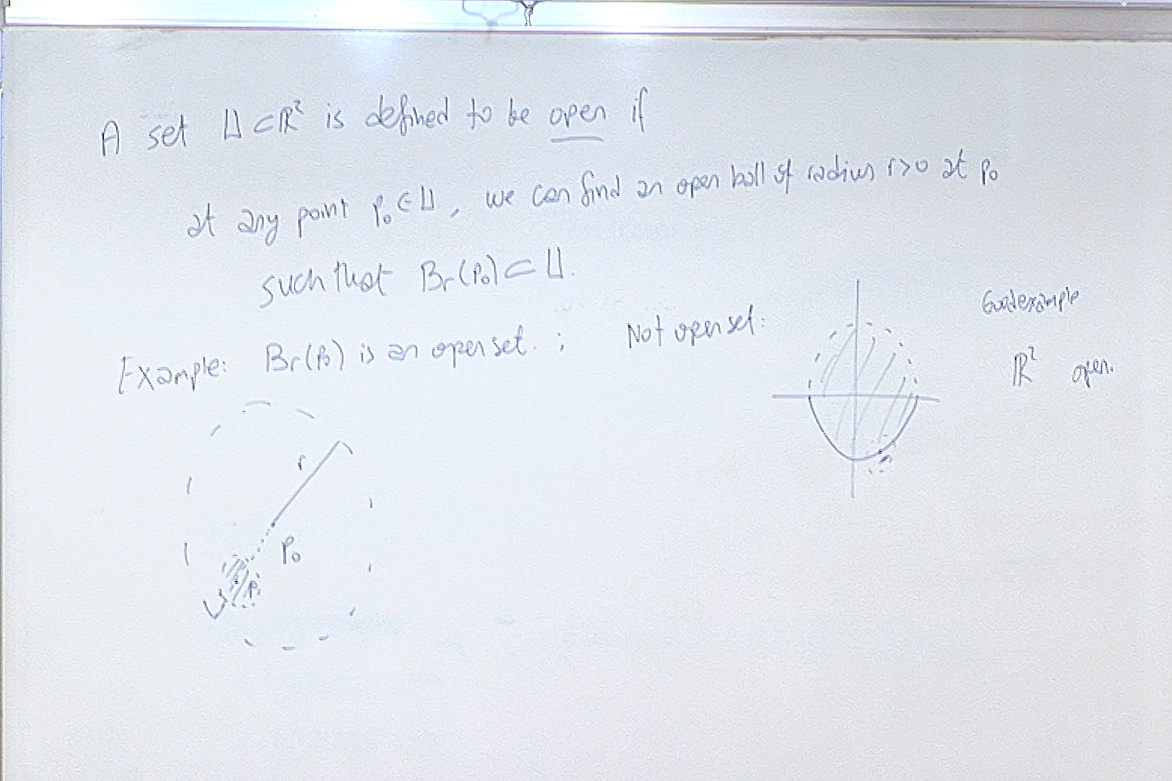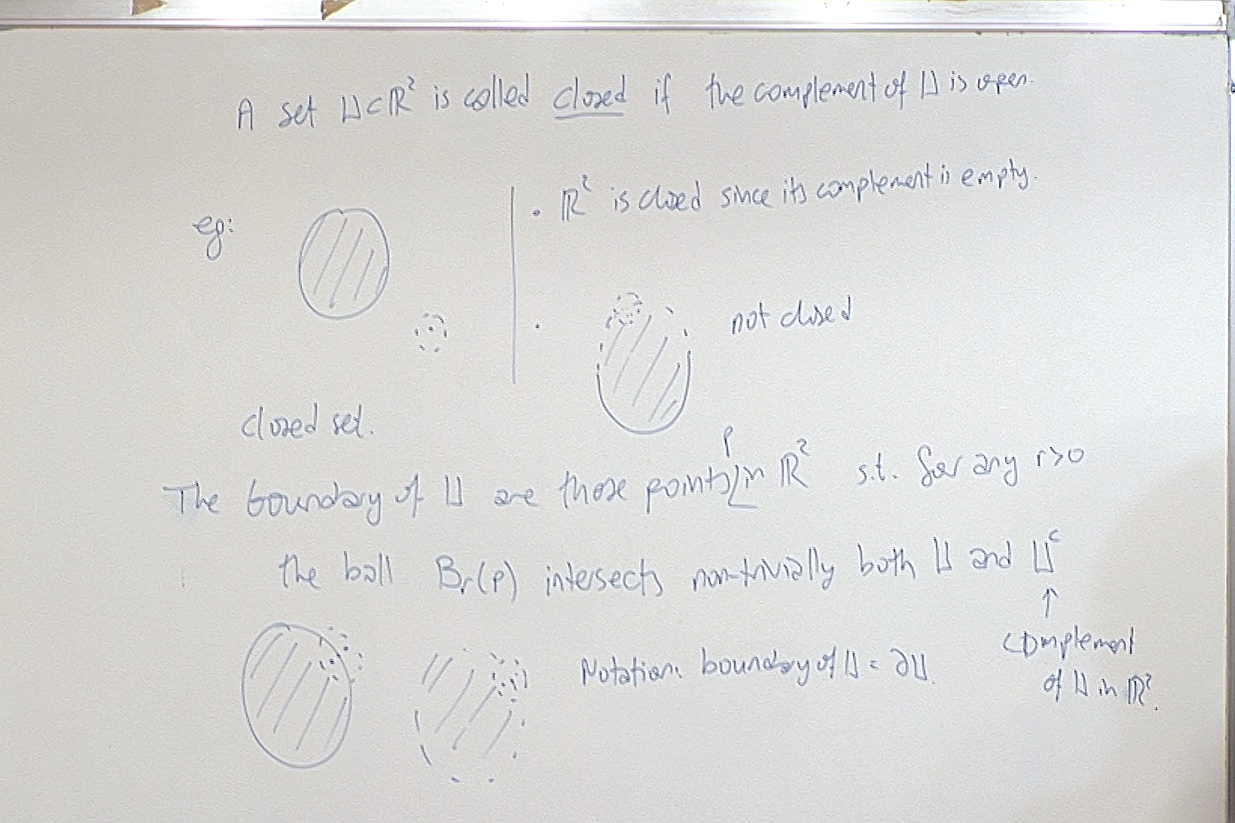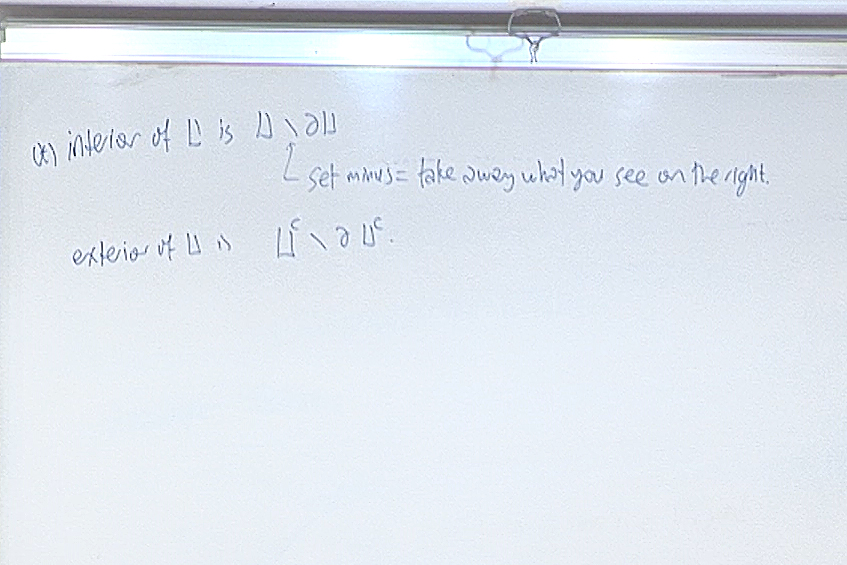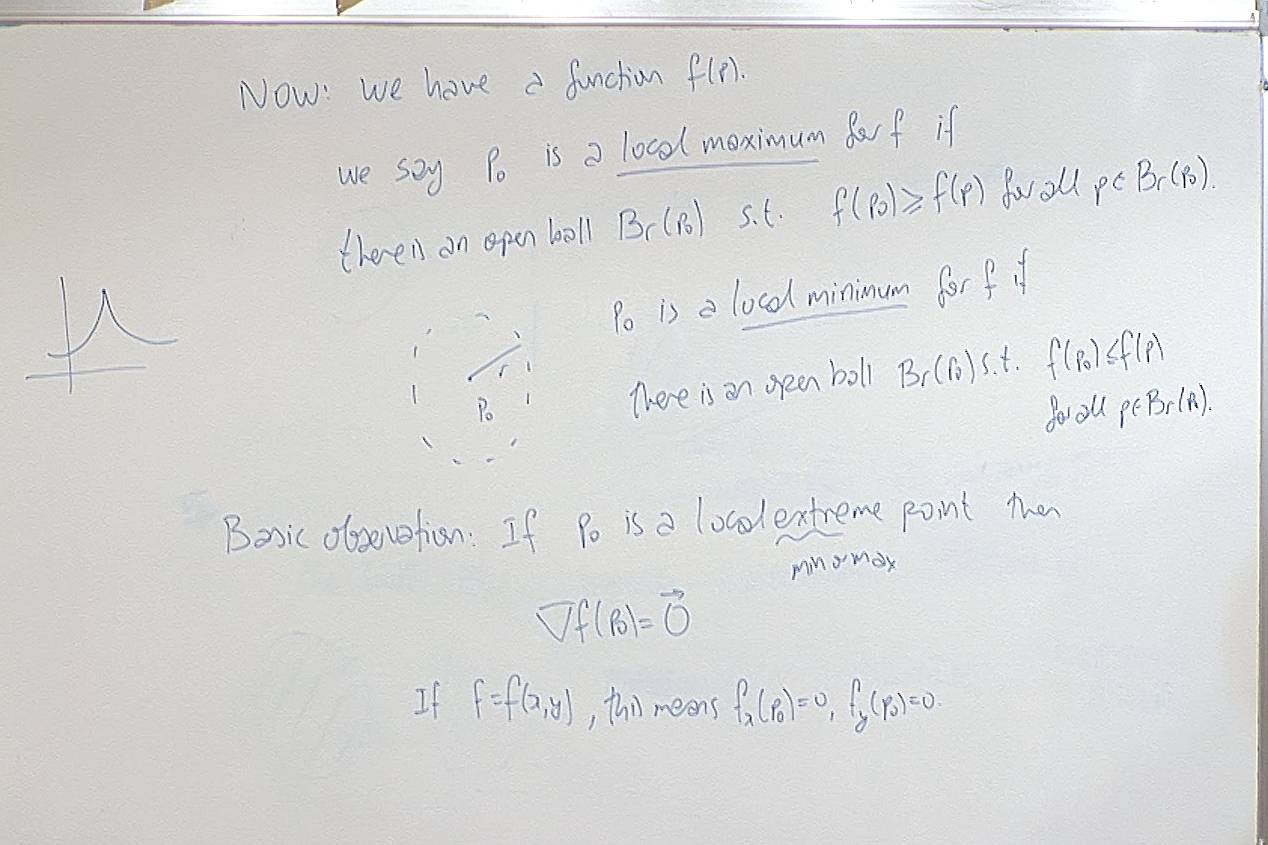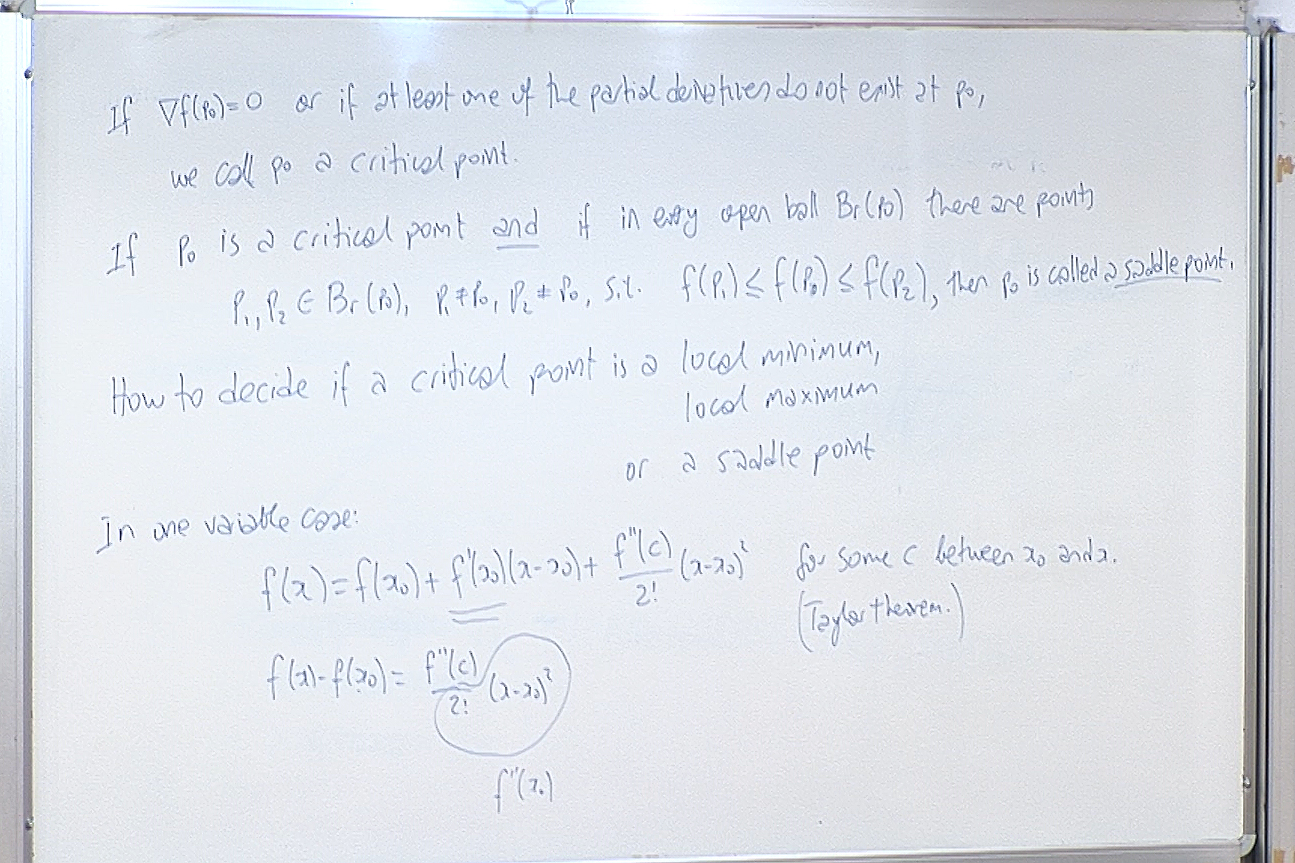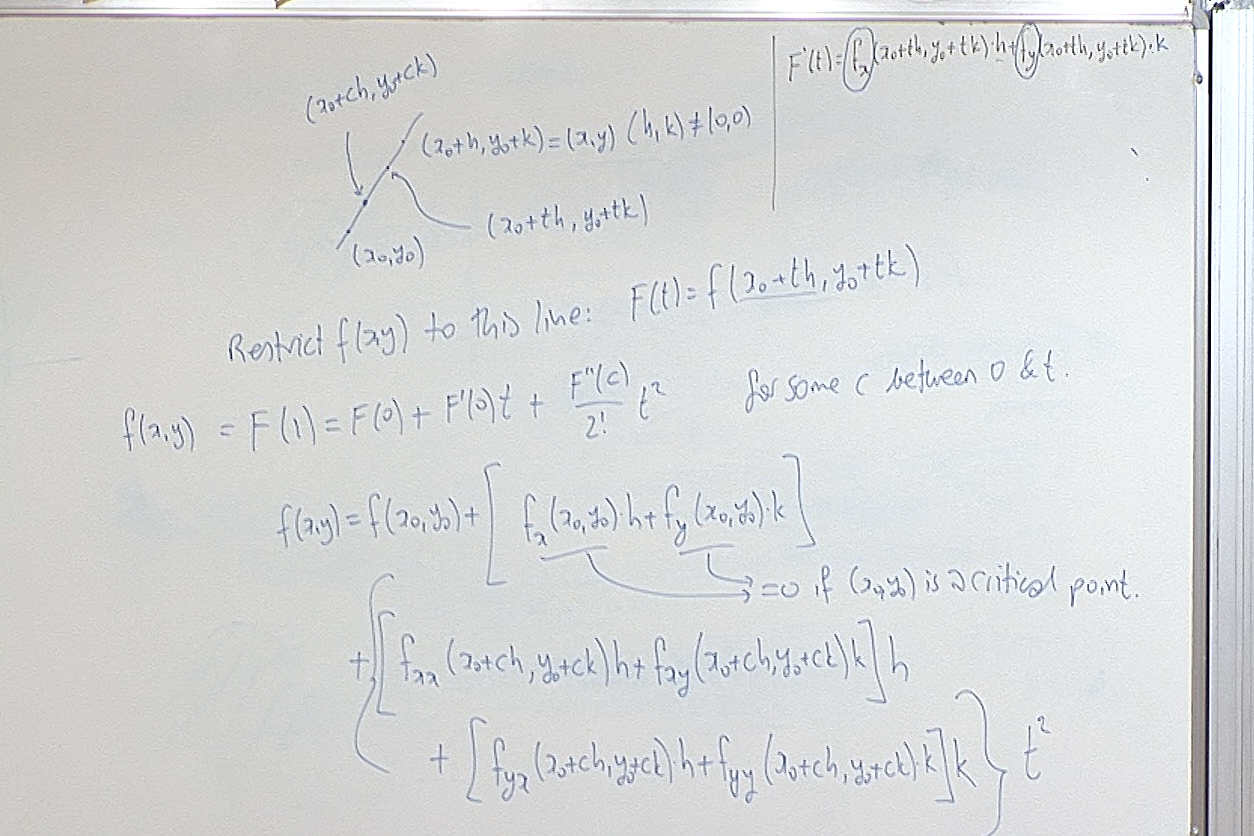The last equation starting with $f(x,y)=\cdots$ is actually the Taylor theorem applied to $F(t)=f(x_0+th,y_0+tk)$ at $t=0$. Here $c$ is some number between $t$ and $0$, whose existence is predicted by Taylor's theorem. We are going to give a proof of this theorem at the beginning of the next class.

For questions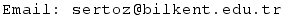Previous Screenshot       Next Screenshot